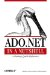# 8.3 Creating Expression Columns

### 8.3 Creating Expression Columns

An expression column can be created to perform calculations on columns in the row or aggregations on a collection of rows. No data is actually stored in the column, and the expression value is evaluated when the column is read. Setting the Expression property to anything other than an empty string automatically sets the ReadOnly property of the column to true . If the expression isn't valid, an EvaluateException is raised.

` // calculate the extended price for the the order detail line DataColumn col1 = new DataColumn("Quantity", typeof(System.Int32)); DataColumn col2 = new DataColumn("Price", typeof(System.Decimal)); // create a column calculating the extended price DataColumn col3 = new DataColumn("ExtendedPrice", typeof(System.Decimal)); Col3.Expression = "Quantity * Price"; `